• 几种聚类算法对比实验 聚类方法是属于无标签的无监督学习方法。其他常见的无监督学习还有密度估计，异常检测等。聚类就是对大量未知标注的数据集，按照数据的内在相似性将数据集划分为多个类别（在聚类算法中称为...
几种聚类算法的对比实验
聚类方法是属于无标签的无监督学习方法。其他常见的无监督学习还有密度估计，异常检测等。聚类就是对大量未知标注的数据集，按照数据的内在相似性将数据集划分为多个类别（在聚类算法中称为簇），使类别内的数据相似度高，二类别间的数据相似度低，我们可以使用聚类分析从我们的数据中获得一些有价值的见解，本文我们将研究几种常见的聚类算法，并讨论他们的优缺点。
kmeans
from copy import deepcopy
import numpy as np
import pandas as pd
import matplotlib
matplotlib.use('TkAgg')
from matplotlib import pyplot as plt

plt.rcParams['figure.figsize'] = (16, 9)
plt.style.use('ggplot')

# 导入数据集
# print(data.shape)

# 将csv文件中的数据转换为二维数组
f1 = data['V1'].values
f2 = data['V2'].values

# 按行的方式计算两个坐标点之间的距离
def dist(a, b, ax=1):
return np.linalg.norm(a - b, axis=ax)
X = np.array(list(zip(f1, f2)))

# 设定分区数
k = 4
# 随机获得中心点的X轴坐标
C_x = np.random.randint(0, np.max(X)-20, size=k)
# 随机获得中心点的Y轴坐标
C_y = np.random.randint(0, np.max(X)-20, size=k)
C = np.array(list(zip(C_x, C_y)), dtype=np.float32)
# 用于保存中心点更新前的坐标
C_old = np.zeros(C.shape)
print(C)
# 用于保存数据所属中心点
clusters = np.zeros(len(X))
# 迭代标识位，通过计算新旧中心点的距离
iteration_flag = dist(C, C_old, 1)

fig, ax = plt.subplots()
plt.scatter(f1, f2, c='black', s=6)
ax.scatter(C[:, 0], C[:, 1], marker='*', s=200, c='blue')

tmp = 1
# 若中心点不再变化或循环次数不超过20次(此限制可取消)，则退出循环
while iteration_flag.any() != 0 and tmp < 20:
# 循环计算出每个点对应的最近中心点
for i in range(len(X)):
# 计算出每个点与中心点的距离
distances = dist(X[i], C, 1)
# print(distances)
# 记录0 - k-1个点中距离近的点
cluster = np.argmin(distances)
# 记录每个样例点与哪个中心点距离最近
clusters[i] = cluster

# 采用深拷贝将当前的中心点保存下来
# print("the distinct of clusters: ", set(clusters))
C_old = deepcopy(C)
# 从属于中心点放到一个数组中，然后按照列的方向取平均值
for i in range(k):
points = [X[j] for j in range(len(X)) if clusters[j] == i]
# print(points)
# print(np.mean(points, axis=0))
C[i] = np.mean(points, axis=0)

# 计算新旧节点的距离
print('循环第%d次' % tmp)
tmp = tmp + 1
iteration_flag = dist(C, C_old, 1)
print("新中心点与旧点的距离：", iteration_flag)

# 最终结果图示
colors = ['r', 'g', 'b', 'y', 'c', 'm']
fig, ax = plt.subplots()
# 不同的子集使用不同的颜色
for i in range(k):
points = np.array([X[j] for j in range(len(X)) if clusters[j] == i])
ax.scatter(points[:, 0], points[:, 1], s=7, c=colors[i])
ax.scatter(C[:, 0], C[:, 1], marker='*', s=200, c='black')

plt.show()


优点：

简单直观，抑郁理解实现；
复杂度相对比较低，在K不是很大的情况下，Kmeans的计算时间相对很短；
Kmean会产生紧密度比较高的簇，反映了簇内样本围绕质心的紧密程度的一种算法。
缺点：
很难预测到准确的簇的数目；
对初始值设置很敏感（Kmeans++）；
Kmeans主要发现圆形或者球形簇，对不同形状和密度的簇效果不好；

LVQ
# -*- coding: utf-8 -*-
"""
Created on Tue Jan 29 20:22:18 2019

@author: zmddzf
"""
import numpy as np
import random
from tqdm import tqdm
import matplotlib.pyplot as plt

class LVQ:
"""
学习向量化算法实现
attributes:
train:LVQ
predict: 预测一个样本所属的簇
"""

def __init__(self, D, T, lr, maxEpoch):
"""
初始化LVQ, 构造器
:param D: 训练集, 格式为[[array, label],...]
:param T: 原型向量类别标记
:param lr: 学习率，0-1之间
:param maxEpoch: 最大迭代次数
"""
self.D = D
self.T = T
self.lr = lr
self.maxEpoch = maxEpoch
self.P = []
# 初始化原型向量，随机选取
for t in T:
while True:
p = random.choice(self.D)
if p != t:
pass
else:
self.P.append(p)
break

def __dist(self, p1, p2):
"""
私有属性，计算距离
:param p1: 向量1
:param p2: 向量2
:return dist: 距离
"""
dist = np.linalg.norm(p1 - p2)
return dist

def train(self):
"""
训练LVQ
:return self.P: 训练后的原型向量
"""
for epoch in tqdm(range(self.maxEpoch)):
x = random.choice(self.D)  # 从训练集随机选取样本
dist = []
for p in self.P:
dist.append(self.__dist(p, x))  # 计算距离列表

t = self.P[dist.index(min(dist))]  # 确定对应最小距离原型向量的类别
if t == x:
# 若类别一致, 则靠拢
self.P[dist.index(min(dist))] = self.P[dist.index(min(dist))] + self.lr * (
x - self.P[dist.index(min(dist))])
else:
# 若类别不同, 则远离
self.P[dist.index(min(dist))] = self.P[dist.index(min(dist))] - self.lr * (
x - self.P[dist.index(min(dist))])
return self.P

def predict(self, x):
"""
预测样本所属的簇
:param x: 样本向量
:return label: 样本的分类结果
"""
dist = []
for p in self.P:
dist.append(self.__dist(p, x))
label = self.P[dist.index(min(dist))]
return label

# 生成实验数据集，数据集是两个正态分布二维点集
mu1 = 2;
sigma1 = 1
mu2 = 4;
sigma2 = 1
# 生成第一个正态分布
samples1 = np.array([np.random.normal(mu1, sigma1, 50), np.random.normal(mu1, sigma1, 50)])
samples1 = samples1.T.tolist()
label1 = [1 for i in range(50)]
# 生成第二个正态分布
samples2 = np.array([np.random.normal(mu2, sigma2, 50), np.random.normal(mu2, sigma2, 50)])
samples2 = samples2.T.tolist()
label2 = [0 for i in range(50)]
# 合并生成数据集
samples = samples1 + samples2
labels = label1 + label2

# 修改数据格式
data = []
for s, l in zip(samples, labels):
data.append([np.array(s), l])

# 开始训练
lvq = LVQ(data, [0, 1], 0.1, 5000)
vector = lvq.train()

# 使用lvq分类
prediction = []
for i in data:
prediction.append(lvq.predict(i))

# 计算accuracy
accuracy = 0
for pred, label in zip(prediction, labels):
if pred == label:
accuracy += 1
accuracy = accuracy / len(data)
print("accuracy of LVQ:", accuracy)

# 画图展示原型向量和散点
plt.figure(figsize=(15, 10))
plt.scatter(np.array(samples).T, np.array(samples).T, c=labels)
plt.scatter(vector, vector, marker='*', s=300)
plt.scatter(vector, vector, marker='*', s=300)

plt.show()


LVQ算法其实也是一种基于竞争的学习，这点和无监督的SOM算法挺像的。LVD算法可以被视为一种网络，由输入层、竞争层、输出层组成。输入层很容易理解，就是接受样本的输入；竞争层可以被视为神经元之间的竞争，也就是原型向量之间的竞争，离得最近的神经元（原型向量）获胜，赢者通吃（winner-take-all）；输出层负责输出分类结果。不论是如何理解这个算法，其实本质都是一样的，也就是同类靠拢、异类远离。
高斯混合聚类
import matplotlib.pyplot as plt
import numpy as np
import math

# 原始数据
x = [0.697, 0.774, 0.634, 0.608, 0.556, 0.403, 0.481, 0.437, 0.666, 0.243,
0.245, 0.343, 0.639, 0.657, 0.360, 0.593, 0.719, 0.359, 0.339, 0.282,
0.748, 0.714, 0.483, 0.478, 0.525, 0.751, 0.532, 0.473, 0.725, 0.446]

y = [0.460, 0.376, 0.264, 0.318, 0.215, 0.237, 0.149, 0.211, 0.091, 0.267,
0.057, 0.099, 0.161, 0.198, 0.370, 0.042, 0.103, 0.188, 0.241, 0.257,
0.232, 0.346, 0.312, 0.437, 0.369, 0.489, 0.472, 0.376, 0.445, 0.459]

# 矩阵测试
def test_matrix():
sigma = np.mat([[0.2, 0.1], [0.0, 0.1]])
sigma_Trans = sigma.T
sigma_inverse = sigma.I
print("sigma: {}".format(sigma))
print("sigma Inverse: {}".format(sigma_inverse))
print("sigma Transform: {}".format(sigma_Trans))

def gauss_density_probability(n, x, mu, sigma):
sigma_det = np.linalg.det(sigma)
divisor = pow(2 * np.pi, n / 2) * np.sqrt(sigma_det)
exp = np.exp(-0.5 * (x - mu) * sigma.I * (x - mu).T)
p = exp / divisor
return p

# 后验概率测试
def test_posterior_probability():
xx = np.mat([[x, y]])
mu_datasets = [np.mat([[x, y]]), np.mat([[x, y]]), np.mat([[x, y]])]
sigma = np.mat([[0.1, 0.0], [0.0, 0.1]])
det = np.linalg.det(sigma)
print("det: {}".format(det))
p_all = []
for mu in mu_datasets:
p = gauss_density_probability(2, xx, mu, sigma)
p = p / 3
p_all.append(p)
p_mean = []
for p in p_all:
p_sum = np.sum(p_all)
p = p / p_sum
p_mean.append(p)
print("probability: {}".format(p_mean))

def posterior_probability(k, steps):

alpha_datasets = [np.mat([1 / k]) for _ in range(k)]
xx = [np.mat([[x[i], y[i]]]) for i in range(len(x))]
mu_rand = np.random.randint(0, 30, (1, k))
print("random: {}".format(mu_rand))
#     mu_datasets = [np.mat([[x[i], y[i]]]) for i in mu_rand]
mu_datasets = [np.mat([[x, y]]), np.mat([[x, y]]), np.mat([[x, y]])]
sigma_datasets = [np.mat([[0.1, 0.0], [0.0, 0.1]]) for _ in range(k)]
#     det = np.linalg.det(sigma_datasets)
for step in range(steps):
p_all = []
# create cluster
classification_temp = locals()
for i in range(k):
classification_temp['cluster_' + str(i)] = []
# 后验概率分组
for j in range(len(x)):
p_group = []
for i in range(k):
mu = mu_datasets[i]
p = gauss_density_probability(2, xx[j], mu, sigma_datasets[i])

p = p * alpha_datasets[i].getA()
p_group.append(p)
p_sum = np.sum(p_group)
# 取最大后验概率
max_p = max(p_group)
max_index = p_group.index(max_p)
# 最大后验概率聚类
for i in range(k):
if i == max_index:
classification_temp['cluster_' + str(max_index)].append(j)

p_group = [p_group[i] / p_sum for i in range(len(p_group))]
p_all.append(p_group)

# 更新 mu, sigma, alpha
mu_datasets = []
sigma_datasets = []
alpha_datasets = []

for i in range(k):
mu_temp_numerator = 0
mu_temp_denominator = 0
sigma_temp = 0
alpha_temp = 0
mu_numerator = [p_all[j][i] * xx[j] for j in range(len(x))]
for mm in mu_numerator:
mu_temp_numerator += mm

mu_denominator = [p_all[j][i] for j in range(len(x))]
for nn in mu_denominator:
mu_temp_denominator += nn

mu_dataset = mu_temp_numerator / mu_temp_denominator
mu_datasets.append(mu_dataset)

sigma = [p_all[j][i].getA() * (xx[j] - mu_dataset).T * (xx[j] - mu_dataset) for j in range(len(x))]
for ss in sigma:
sigma_temp += ss
sigma_dataset = sigma_temp / mu_temp_denominator
sigma_datasets.append(sigma_dataset)

alpha_new = [p_all[j][i] for j in range(len(x))]
for alpha_nn in alpha_new:
alpha_temp += alpha_nn
alpha_dataset = alpha_temp / len(x)
alpha_datasets.append(alpha_dataset)
return p_all, mu_datasets, sigma_datasets, alpha_datasets, classification_temp

def cluster_visiualization(k, steps):
post_probability, mu_datasets, sigma_datasets, alpha_datasets, classification_temp = posterior_probability(k, steps)
plt.figure(figsize=(8, 8))
markers = ['.', 's', '^', '<', '>', 'P']
plt.xlim(0.1, 0.9)
plt.ylim(0, 0.9)
plt.grid()
plt.scatter(x, y, color='r')

plt.figure(figsize=(8, 8))
for i in range(k):
# 依据聚类获取对应数据，并显示
xx = [x[num] for num in classification_temp['cluster_' + str(i)]]
yy = [y[num] for num in classification_temp['cluster_' + str(i)]]

plt.xlim(0.1, 0.9)
plt.ylim(0, 0.9)
plt.grid()
plt.scatter(xx, yy, marker=markers[i])
plt.savefig("./images/gauss_cluster.png", format="png")

if __name__ == "__main__":
cluster_visiualization(3, 100)


算法本身不复杂，可能涉及到矩阵求导的部分会麻烦一点。西瓜数据集太小了，收敛非常快。然后，这个算法同样对于初值敏感。
DBSCAN
import numpy as np
from sklearn.cluster import DBSCAN
from sklearn import metrics
from sklearn.datasets.samples_generator import make_blobs
from sklearn.preprocessing import StandardScaler

# #############################################################################
# 产生样本数据
centers = [[1, 1], [-1, -1], [1, -1]]  # 生成聚类中心点
X, labels_true = make_blobs(n_samples=750, centers=centers, cluster_std=0.4,random_state=0) # 生成样本数据集

X = StandardScaler().fit_transform(X) # StandardScaler作用：去均值和方差归一化。且是针对每一个特征维度来做的，而不是针对样本。

# #############################################################################
# 调用密度聚类  DBSCAN
db = DBSCAN(eps=0.3, min_samples=10).fit(X)
# print(db.labels_)  # db.labels_为所有样本的聚类索引，没有聚类索引为-1
# print(db.core_sample_indices_) # 所有核心样本的索引
core_samples_mask = np.zeros_like(db.labels_, dtype=bool)  # 设置一个样本个数长度的全false向量
labels = db.labels_

# 获取聚类个数。（聚类结果中-1表示没有聚类为离散点）
n_clusters_ = len(set(labels)) - (1 if -1 in labels else 0)

# 模型评估
print('估计的聚类个数为: %d' % n_clusters_)
print("同质性: %0.3f" % metrics.homogeneity_score(labels_true, labels))  # 每个群集只包含单个类的成员。
print("完整性: %0.3f" % metrics.completeness_score(labels_true, labels))  # 给定类的所有成员都分配给同一个群集。
print("V-measure: %0.3f" % metrics.v_measure_score(labels_true, labels))  # 同质性和完整性的调和平均
print("轮廓系数: %0.3f" % metrics.silhouette_score(X, labels))

# #############################################################################
# Plot result
import matplotlib.pyplot as plt

# 使用黑色标注离散点
unique_labels = set(labels)
colors = [plt.cm.Spectral(each) for each in np.linspace(0, 1, len(unique_labels))]
for k, col in zip(unique_labels, colors):
if k == -1:  # 聚类结果为-1的样本为离散点
# 使用黑色绘制离散点
col = [0, 0, 0, 1]

class_member_mask = (labels == k)  # 将所有属于该聚类的样本位置置为true

plt.plot(xy[:, 0], xy[:, 1], 'o', markerfacecolor=tuple(col),markeredgecolor='k', markersize=14)

plt.plot(xy[:, 0], xy[:, 1], 'o', markerfacecolor=tuple(col),markeredgecolor='k', markersize=6)

plt.title('Estimated number of clusters: %d' % n_clusters_)
plt.show()

DBSCAN的主要优点有：
1） 可以对任意形状的稠密数据集进行聚类，相对的，K-Means之类的聚类算法一般只适用于凸数据集。
2） 可以在聚类的同时发现异常点，对数据集中的异常点不敏感。
3） 聚类结果没有偏倚，相对的，K-Means之类的聚类算法初始值对聚类结果有很大影响。
DBSCAN的主要缺点有：
1）如果样本集的密度不均匀、聚类间距差相差很大时，聚类质量较差，这时用DBSCAN聚类一般不适合。
2）如果样本集较大时，聚类收敛时间较长，此时可以对搜索最近邻时建立的KD树或者球树进行规模限制来改进。
3） 调参相对于传统的K-Means之类的聚类算法稍复杂，主要需要对距离阈值ϵ，邻域样本数阈值MinPts联合调参，不同的参数组合对最后的聚类效果有较大影响。
AGNES
 #-*- coding:utf-8 -*-

import math
import pylab as pl

#数据集：每三个是一组分别是西瓜的编号，密度，含糖量
data = """
1,0.697,0.46,2,0.774,0.376,3,0.634,0.264,4,0.608,0.318,5,0.556,0.215,
6,0.403,0.237,7,0.481,0.149,8,0.437,0.211,9,0.666,0.091,10,0.243,0.267,
11,0.245,0.057,12,0.343,0.099,13,0.639,0.161,14,0.657,0.198,15,0.36,0.37,
16,0.593,0.042,17,0.719,0.103,18,0.359,0.188,19,0.339,0.241,20,0.282,0.257,
21,0.748,0.232,22,0.714,0.346,23,0.483,0.312,24,0.478,0.437,25,0.525,0.369,
26,0.751,0.489,27,0.532,0.472,28,0.473,0.376,29,0.725,0.445,30,0.446,0.459"""

#数据处理 dataset是30个样本（密度，含糖量）的列表
a = data.split(',')
dataset = [(float(a[i]), float(a[i+1])) for i in range(1, len(a)-1, 3)]

#计算欧几里得距离,a,b分别为两个元组
def dist(a, b):
return math.sqrt(math.pow(a-b, 2)+math.pow(a-b, 2))

#dist_min
def dist_min(Ci, Cj):
return min(dist(i, j) for i in Ci for j in Cj)
#dist_max
def dist_max(Ci, Cj):
return max(dist(i, j) for i in Ci for j in Cj)
#dist_avg
def dist_avg(Ci, Cj):
return sum(dist(i, j) for i in Ci for j in Cj)/(len(Ci)*len(Cj))

#找到距离最小的下标
def find_Min(M):
min = 1000
x = 0; y = 0
for i in range(len(M)):
for j in range(len(M[i])):
if i != j and M[i][j] < min:
min = M[i][j];x = i; y = j
return (x, y, min)

#算法模型：
def AGNES(dataset, dist, k):
#初始化C和M
C = [];M = []
for i in dataset:
Ci = []
Ci.append(i)
C.append(Ci)
for i in C:
Mi = []
for j in C:
Mi.append(dist(i, j))
M.append(Mi)
q = len(dataset)
#合并更新
while q > k:
x, y, min = find_Min(M)
C[x].extend(C[y])
C.remove(C[y])
M = []
for i in C:
Mi = []
for j in C:
Mi.append(dist(i, j))
M.append(Mi)
q -= 1
return C
#画图
def draw(C):
colValue = ['r', 'y', 'g', 'b', 'c', 'k', 'm']
for i in range(len(C)):
coo_X = []    #x坐标列表
coo_Y = []    #y坐标列表
for j in range(len(C[i])):
coo_X.append(C[i][j])
coo_Y.append(C[i][j])
pl.scatter(coo_X, coo_Y, marker='x', color=colValue[i%len(colValue)], label=i)

pl.legend(loc='upper right')
pl.show()

C = AGNES(dataset, dist_avg, 3)
draw(C)


AGNES算法比较简单，但一旦一组对象被合并，下一步的处理将在新生成的簇上进行。已做处理不能撤消，聚类之间也不能交换对象。增加新的样本对结果的影响较大。
假定在开始的时候有nn个簇，在结束的时候有11个簇，因此在主循环中有nn次迭代，在第ii次迭代中，我们必须在n−i+1n−i+1个簇中找到最靠近的两个进行合并。另外算法必须计算所有对象两两之间的距离，因此这个算法的复杂度为 O(n2)O(n2)，该算法对于nn很大的情况是不适用的。


展开全文机器学习
• 0 写在前面（数据集和源代码）本文章...一共有四个代码文件，分别是Kmeans、Kmeans++、Birch和KNN算法，四个算法对同一个数据集聚类分析进行对比试验。（本代码是本人自己书写，全部可用！）1 引言近年来，机器学习...
0 写在前面（数据集和源代码）本文章涉及到的数据集合所有代码均上传在此处：https://download.csdn.net/download/zhouzhuo_csuft/10494273；点击此处直接打开链接；一共有四个代码文件，分别是Kmeans、Kmeans++、Birch和KNN算法，四个算法对同一个数据集聚类分析进行对比试验。（本代码是本人自己书写，全部可用！）1 引言近年来，机器学习已成为计算机前沿中火热的研究点之一。我国政府也将机器学习纳入了国家级战略。目前，机器学习已广泛用于各种数据挖掘、模式识别、语音识别及图像处理等各领域。本文以机器学习中四个经典的聚类算法进行对比介绍和对比实验，得出四个算法对相同实验数据集的聚类效果。2聚类算法聚类在学术界并没有一个确切的定义，此处给出1974年Everitt对聚类的定义：一个类簇内的实体是相似的,不同类簇的实体是不相似的;一个类簇是测试空间中点的会聚,同一类簇的任意两个点间的距离小于不同类簇的任意两个点间的距离;类簇可以描述为一个包含密度相对较高的点集的多维空间中的连通区域,它们借助包含密度相对较低的点集的区域与其他区域(类簇)相分离。简单地说，聚类是根据其自身特征将数据集进行划分成若干类的过程，划分的结果是相同类哈哈内数据相似度尽可能大、不同类间数据相似度尽可能小，从而发现数据集的内在结构。聚类在不同应用领域有不同的特殊要求，聚类算法的典型性能要求有以下几个方面：（1）伸缩性（2）兼容性（3）有效处理噪声数据（4）能处理基于约束的聚类（5）可解释性和可用性典型的聚类过程主要包括数据（样本）准备、特征选择和特征提取、相似度计算、聚类、对聚类结果进行有效性评估。聚类过程：（1）数据准备：包括特征标准化和降维。（2）特征选择，从最初的特征中选取最有效的特征，并将其存储于向量中。（3）特征提取：通过对所选择的特征进行转换形成新的突出特征。（4）聚类：首先选择合适特征类型的某种离函数 ( 或构造新的距离函数 ) 进行接近程度的度量，而后执行聚类或分组。 .（5）聚类结果评估 : 是指对聚类结果进行评估 . 评估主要有 3 种 : 外部有效性评估、内部有效性评估和相关性测试评估。3 典型聚类及其变种算法介绍3.1 K-Means聚类3.2.1 K-Means聚类介绍1967 年 ,MacQueen 首次提出了 K 均值聚类算法 (K-Means 算法 )。 迄今为止 , 很多聚类任务都选择该经典算法。该算法的核心思想是找出 k个聚类中心c1 ,c2 ,…,ck使得每一个数据点 xi 和与其最近的聚类中心 cv 的平方距离和被最小化 ( 该平方距离和被称为偏差 D)。3.1.2 K-means聚类算法步骤（1）初始化，随机指定 K 个聚类中心 (c1 ,c2 ,…,ck );（2）分配 xi, 对每一个样本 xi，找到离它最近的聚类中心 cv，并将其分配到 cv 所标明类;（3）修正 c w，将每一个 cw 移动到其标明的类的中心；（4）计算偏差                              ；（5）判断D是否收敛，如果D收敛，则return(c1,c2,……,ck)，并终止本算法；否则返回步骤2。3.1.3 K-means 算法的优点与不足优点: 能对大型数据集进行高效分类, 其计算复杂性为 O(tkmn), 其中 ,t 为迭代次数，k为聚类数，m 为特征属性数，n 为待分类的对象数，通常k,m,t<<n. 在对大型数据集聚类时，K-means 算法比层次聚类算法快得多。 不足: 通常会在获得一个局部最优值时终止；仅适合对数值型数据聚类；只适用于聚类。3.2 K-Means++算法3.2.1 K-Means++简介由于 K-means 算法的分类结果会受到初始点的选取而有所区别，因此后来有人针对这个问题对K-Means算法进行改进，于是产生了 K-means++算法 。K-Means++算法只是对初始点的选择有改进而已，其他步骤都一样。初始质心选取的基本思路就是，初始的聚类中心之间的相互距离要尽可能的远。3.2.2  K-Means++算法步骤（1）从数据集中随机选取一个样本作为初始聚类中心c1;（2）首先计算每个样本与当前已有聚类中心之间的最短距离（即最近的一个聚类中心的距离），用D(x)表示；接着计算每个样本被选为下一个聚类中心的概率  。按照轮盘法选出下一个聚类中心;（3）重复第2步，直到选择出共K个聚类中心；（4）后面与K-Means聚类算法的2-4步相同。3.3 BIRCH聚类算法3.3.1 BIRCH聚类简介　BIRCH的全称是利用层次方法的平衡迭代规约和聚类（Balanced Iterative Reducing and Clustering UsingHierarchies），它利用了一个类似于B+树的聚类特征树(ClusteringFeature Tree，简称CF Tree)快速的聚类。如图1所示，这颗树的每一个节点是由若干个聚类特征(Clustering Feature，简称CF)组成。每个节点包括叶子节点都有若干个CF，而内部节点的CF有指向孩子节点的指针，所有的叶子节点用一个双向链表链接起来。不同于K-Means算法，BIRCH算法可以不用输入类别数K值。如果不输入K值，则最后的CF元组的组数即为最终的K，否则会按照输入的K值对CF元组按距离大小进行合并。一般来说，BIRCH算法适用于样本量较大的情况，除了聚类还可以额外做一些异常点检测和数据初步按类别规约的预处理。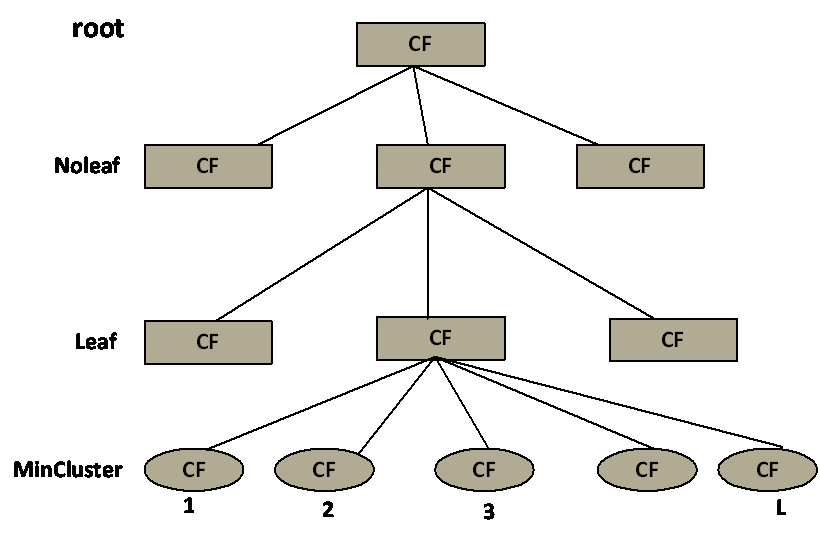图 1 CF Tree模型3.3.2 BIRCH聚类算法步骤（1）扫描所有数据，建立初始化的CF树，把稠密数据分成簇，稀疏数据作为孤立点对待；（2）这个阶段是可选的，阶段（3）的全局或半全局聚类算法有着输入范围的要求，以达到速度与质量的要求，所以此阶段在阶段步骤（1）的基础上，建立一个更小的CF树；（3）补救由于输入顺序和页面大小带来的分裂，使用全局/半全局算法对全部叶节点进行聚类（4）这个阶段也是可选的，把阶段（3）的中心点作为种子，将数据点重新分配到最近的种子上，保证重复数据分到同一个簇中，同时添加簇标签。3.3.4 BIRCH聚类的优缺点主要优点有：（1）节约内存，所有的样本都在磁盘上，CF Tree仅仅存了CF节点和对应的指针。（2）聚类速度快，只需要一遍扫描训练集就可以建立CF Tree，CF Tree的增删改都很快。（3）可以识别噪音点，还可以对数据集进行初步分类的预处理主要缺点有：（1）由于CF Tree对每个节点的CF个数有限制，导致聚类的结果可能和真实的类别分布不同.（2）对高维特征的数据聚类效果不好。此时可以选择Mini Batch K-Means（3）如果数据集的分布簇不是类似于超球体，或者说不是凸的，则聚类效果不好。3.4 KNN聚类算法3.4.1 KNN聚类简介KNN（K-Nearest Neighbor），代表 k 个最近邻分类法，通过k个与之最相近的历史记录的组合来辨别新的记录。KNN 是一个众所周知的统计方法，在过去的 40 年里在模式识别中集中地被研究。KNN 在早期的研究策略中已被应用于文本分类，是基准 Reuters 主体的高操作性的方法之一。其它方法，如 LLSF、决策树和神经网络等。3.4.2 KNN聚类算法步骤（1）准备数据，对数据进行预处理；（2） 选用合适的数据结构存储训练数据和测试元组；（3）设定参数，如元组数目k；（4）维护一个大小为k的按距离由大到小的优先级队列，用于存储最近邻训练元组。随机从训练元组中选取k个元组作为初始的最近邻元组，分别计算测试元组到这k个元组的距离，将训练元组标号和距离存入优先级队列；（5）遍历训练元组集，计算当前训练元组与测试元组的距离，将所得距离L 与优先级队列中的最大距离Lmax；（6）进行比较。若L>=Lmax，则舍弃该元组，遍历下一个元组。若L < Lmax，删除优先级队列中最大距离的元组，将当前训练元组存入优先级队列；（7）遍历完毕，计算优先级队列中k 个元组的多数类，并将其作为测试元组的类别；（8） 测试元组集测试完毕后计算误差率，继续设定不同的k值重新进行训练，最后取误差率最小的k 值。3.4.3KNN聚类的优缺点主要优点有（1）简单，易于理解，无需建模与训练，易于实现；（2）适合对稀有事件进行分类；（3）适合与多分类问题，例如根据基因特征来判断其功能分类，KNN比SVM的表现要好。主要缺点有：（1）惰性算法，内存开销大，对测试样本分类时计算量大，性能较低；（2）可解释性差，无法给出决策树那样的规则。4 实验4.1 实验环境本实验拟将对第二节中的4个算法进行测试，实验系统采用Ubuntu16.04TLS版本（也可以用windows下pycharm等IDE），内存8G，CPU为4核心AMD芯片（两个），主频2.4GHZ。实验代码使用Python2.7.2版本。4.2实验测试      为测试4个算法的聚类效果，实验将使用4个算法对同一个数据集进行聚类测试。该数据集是一个存放80个二维坐标点的文本文件，文件每行有两个数据，以制表符分割，分别表示二维坐标的X轴数据和Y轴数据。      经测试，4个算法对数据集聚类的结果图示如图2、图3、图4、图5所示：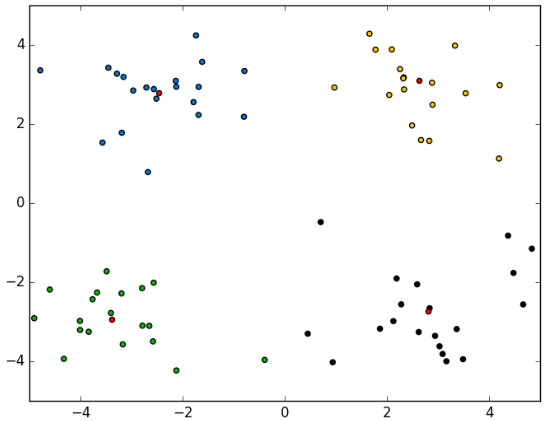图 2 K-Means聚类结果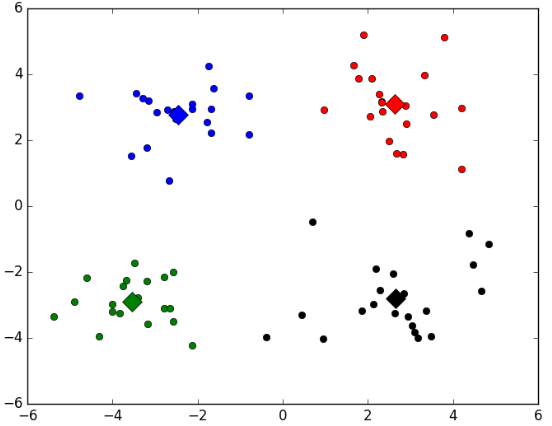图 3 K-Means++聚类结果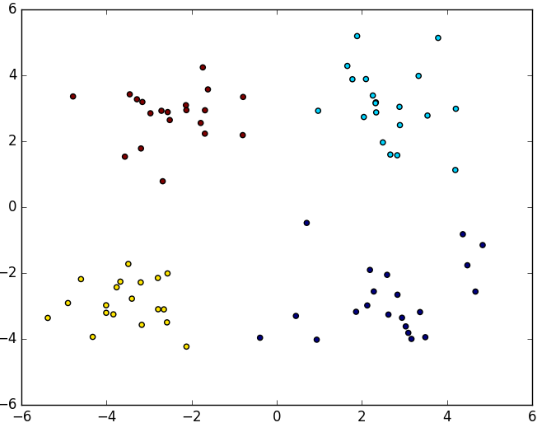图 4 BIRCH聚类结果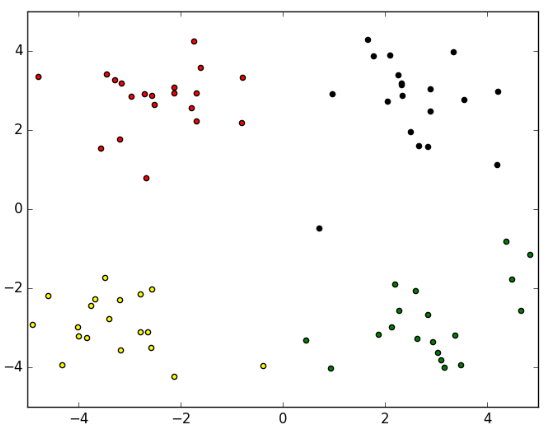图 5 KNN聚类结果4.3 对比结论上述四组图为实验对比，可以看出，四种算法对数据集的分类效果相当，只有在个别点的划分有所不同，即数据集中的(-0.392370  -3.963704)坐标点，在K-Means聚类和KNN聚类中，该点被聚类到左下方簇中，而在K-Means++聚类和BIRCH聚类中，该点被划分到了右下方簇中。5总结      本文首先介绍了聚类算法定义和聚类算法的基本步骤，然后分别对K-Means、K-Means++、BIRCH和KNN四个经典的聚类算法作了详细介绍，最后通过对同一个数据集进行对比实验，得出四个聚类效果。从实验中反映了四个聚类算法对数据集的聚类效果较好。四种聚类算法在本实验中达到了的聚类效果几乎一样，但四类聚类算法在实际生活中仍将有其不同的使用背景，故在实际生活中需要结合特定的实际环境采用不同聚类算法，以达到最好的效果。6参考文献 孙吉贵，刘杰，赵连宇.聚类算法研究[J].软件学报,2008 张建萍,刘希玉.基于聚类分析的 K-means算法研究及应用[J].计算机应用研究,2007,5 杨善林,李永森,胡笑旋,潘若愚.K-means 算法中的 k 值优化问题研究[J].系统工程理论与实践，2006,2 David Arthur and Sergei Vassilvitskii.k-means++:[J].TheAdvantages of Careful Seeding，2007 BIRCH:An Efficient Data 3Clustering Method forVery Large Databases[J].1996张宁,贾自艳,史忠植.使用 KNN算法的文本分类[J].计算机工程，2005.4KNN算法综述,https://wenku.baidu.com/view/d84cf670a5e9856a561260ce.html,2018.5.10 贺玲,吴玲达,蔡益朝.数据挖掘中的聚类算法综述[J].计算机应用研究,2007
展开全文机器学习 Kmeans++ Birch
• #-*-coding:utf-8-*- import numpy as np import matplotlib.pyplot ...可以看出，当聚类数量为3时，比较明显的地方是dbscan可以聚类出形状相似的部分，而且其它的方法都需要指定聚类的簇数
#-*-coding:utf-8-*-
import numpy as np
import matplotlib.pyplot as plt
from sklearn import datasets

x1,y1=datasets.make_circles(n_samples=5000,factor=.6,noise=0.05)

x2,y2=datasets.make_blobs(n_samples=1000,n_features=2,centers=[[1.2,1.2]],cluster_std=[[.1]],random_state=9)

#x3,y3=datasets.make_moons(n_samples=2000,noise=0.05)
#plt.scatter(x3[:,0],x3[:,1],marker='o')
#plt.show()

plt.subplot(3,2,6)#把原始图像放在最后显示
x=np.concatenate((x1,x2))
plt.scatter(x[:,0],x[:,1],marker='o')

plt.subplot(3,2,1)#第一个是kmeans的结果，K为5
from sklearn.cluster import KMeans
result=KMeans(n_clusters=5,random_state=9).fit_predict(x)
plt.scatter(x[:,0],x[:,1],c=result)
#plt.show()
plt.subplot(3,2,2)
from sklearn.cluster import MiniBatchKMeans#第二个与第一个类似，不同的地方是计算距离使用的样本是不同类的抽样数据
result=MiniBatchKMeans(n_clusters=5,random_state=9).fit_predict(x)
plt.scatter(x[:,0],x[:,1],c=result)
#plt.show()
plt.subplot(3,2,3)
from sklearn.cluster import Birch#利用层次方法的平衡迭代和规约
result=Birch(n_clusters=5).fit_predict(x)
plt.scatter(x[:,0],x[:,1],c=result)
'''
plt.subplot(3,2,1)
from sklearn.cluster import AffinityPropagation#吸引子传播，这个计算起来很慢.....
result=AffinityPropagation().fit_predict(x)
plt.scatter(x[:,0],x[:,1],c=result)
plt.show()
'''

plt.subplot(3,2,4)
from sklearn.cluster import DBSCAN#具有噪声的基于密度的聚类方法，不需要指定聚类类别，但需要指定距离和簇最小样本
result=DBSCAN(eps=0.1,min_samples=10).fit_predict(x)
plt.scatter(x[:,0],x[:,1],c=result)

plt.subplot(3,2,5)
from sklearn.cluster import SpectralClustering#谱聚类，计算相似度矩阵，相似距离使用rbf距离
result=SpectralClustering(n_clusters=5).fit_predict(x)
plt.scatter(x[:,0],x[:,1],c=result)
plt.show()

可以看出，当聚类数量为3时，比较明显的地方是dbscan可以聚类出形状相似的部分，而且其它的方法都需要指定聚类的簇数

展开全文• 有一个实验要求对比两种大数据聚类算法的性能，具体的代码也不是由我实现的，我只是改了一部分，主要还是博客大佬们的代码，我这里借用了一下~~ 具体的实验报告和python源码文件在最后位置，提供百度云下载，本文...
在大数据领域这个聚类算法真是起到了十分重要的作用，只有通过有效地聚类才能得到非常直观的结果。
有一个实验要求对比两种大数据聚类算法的性能，具体的代码也不是由我实现的，我只是改了一部分，主要还是博客大佬们的代码，我这里借用了一下~~
具体的实验报告和python源码文件在最后位置，提供百度云下载，本文使用的是K-means算法和层次聚类算法AGNES，原理介绍和实验结果详见百度云提供的报告等
如今大数据的时代，大量的可以获得的数据被保存，用来分析以获得有用的信息，然而数据纷繁杂乱，需要研究者对齐进行一定的划分，将相似的数据放在一起进行分析，这就是所谓的聚类。
聚类就是按照某个特定标准(如距离准则，即数据点之间的距离)把一个数据集分割成不同的类或簇，使得同一个簇内的数据对象的相似性尽可能大，同时不在同一个簇中的数据对象的差异性也尽可能地大，常用的基本的距离计算方式就是欧氏距离。我们可以具体地理解为，聚类后同一类的数据尽可能聚集到一起，不同类数据尽量分离。聚类技术正在蓬勃发展，应用到的领域包括数据挖掘、统计学、机器学习、空间数据库技术、生物学以及市场营销等。各种聚类方法也被不断提出和改进，而不同的方法适合于不同类型的数据，因此对各种聚类方法、聚类效果的比较是一个值得研究的问题。
目前，有大量的聚类算法。而对于具体应用，聚类算法的选择取决于数据的类型、聚类的目的。如果聚类分析被用作描述或探查的工具，可以对同样的数据尝试多种算法，以发现数据可能揭示的结果。主要的聚类算法可以划分为如下几类：划分方法、层次方法、基于密度的方法、基于网格的方法以及基于模型的方法。
K-means聚类算法
K-means是划分方法中较经典的聚类算法之一。由于该算法的效率高，所以在对大规模数据进行聚类时被广泛应用。目前，许多算法均围绕着该算法进行扩展和改进。K-means算法目标是，以k为参数，把n个对象分成k个簇，使簇内具有较高的相似度，而簇间的相似度较低。
K-means算法的处理过程如下：首先，随机地 选择k个对象，每个对象初始地代表了一个簇的平均值或中心;对剩余的每个对象，根据其与各簇中心的距离，将它赋给最近的簇;然后重新计算每个簇的平均值。 这个过程不断重复，直到准则函数收敛。
通常，采用平方误差准则。平方误差准则采用的是数据库中所有对象的平方误差的总和，与是空间中的点和每个簇的的平均值有关。目标是使生成的簇尽可能紧凑独立，使用的距离度量是欧几里得距离,当然也可以用其他距离度量。K-means聚类算法的算法流程如下：
输入：包含n个对象的数据库和簇的数目k；
输出：k个簇，使平方误差准则最小。
步骤：
(1) 任意选择k个对象作为初始的簇中心；
(2) repeat；
(3) 根据簇中对象的平均值，将每个对象(重新)赋予最类似的簇；
(4) 更新簇的平均值，即计算每个簇中对象的平均值；
(5) until不再发生变化。
优点：简单直接（体现在逻辑思路以及实现难度上），易于理解，在低维数据集上有不错的效果（简单的算法不见得就不能得到优秀的效果）。
缺点：对于高维数据（如成百上千维，现实中还不止这么多），其计算速度十分慢，主要是慢在计算距离上（参考欧几里得距离，当然并行化处理是可以的，这是算法实现层面的问题），它的另外一个缺点就是它需要我们设定希望得到的聚类数k，若我们对于数据没有很好的理解，那么设置k值就成了一种估计性的工作，并且会对实验结果造成很大的偏差。
层次聚类算法
根据层次分解的顺序是自底向上的还是自上向下的，层次聚类算法分为凝聚的层次聚类算法和分裂的层次聚类算法。
凝聚型层次聚类的策略是先将每个对象作为一个簇，然后合并这些原子簇为越来越大的簇，直到所有对象都在一个簇中，或者某个终结条件被满足。绝大多数层次聚类属于凝聚型层次聚类，它们只是在簇间相似度的定义上有所不同。四种广泛采用的簇间距离度量方法为：最小距离，最大距离，平均值距离，平均距离。
这里以最小距离的凝聚层次聚类算法流程为例：
(1) 将每个对象看作一类，计算两两之间的最小距离；
(2) 将距离最小的两个类合并成一个新类；
(3) 重新计算新类与所有类之间的距离；
(4) 重复(2)、(3)，直到所有类最后合并成一类。
优点：

距离和规则的相似度容易定义，限制少；
不需要预先制定聚类数；
可以发现类的层次关系（在一些特定领域如生物有很大作用）；
缺点：
计算复杂度太高（考虑并行化）；
奇异值也能产生很大影响；
算法很可能聚类成链状（一层包含着一层）；
算法不需要预定聚类数，但是我们选择哪个层次的聚类作为我们需要的聚类效果，这需要我们按照实际客观情况以及经验来完成，毕竟就凝聚聚类来说，从最底层的每个个体作为一个个体，到最顶层所有个体合并为一个个体，其中的聚类结果可能有许许多多种。当然针对这个问题也有许多解决方案，其中一个常用的就是凝聚到某个程度其聚类之间的距离都大于某个阈值k，就停止凝聚。

具体代码
在实验中，本人先是下载的点集，然后为了测试效率写了一个简单的产生大量点的脚本，具体的实现代码如下（来自网络 进行了小小的更改）,注意需要的库文件，没有的安装一下，还有路径也需要改：
AGNES
#-*- coding:utf-8 -*-
import math
import pylab as pl
import codecs
import re
import datetime

pl.rcParams['axes.unicode_minus'] = False
# 为了显示汉字坐标的

#计算欧式距离,a,b代表两个元组
def calcudistance(a, b):
return math.sqrt(math.pow(a-b, 2)+math.pow(a-b, 2))

# 求出最小距离
def dist_min(Ci, Cj):
return min(calcudistance(i, j) for i in Ci for j in Cj)

# 求出最大距离
def dist_max(Ci, Cj):
return max(calcudistance(i, j) for i in Ci for j in Cj)

#求出平均距离
def dist_avg(Ci, Cj):
return sum(calcudistance(i, j) for i in Ci for j in Cj)/(len(Ci)*len(Cj))

#找到距离最小的下标
def find_Min(M):
min = 1000
x = 0; y = 0
for i in range(len(M)):
for j in range(len(M[i])):
if i != j and M[i][j] < min:
min = M[i][j];x = i; y = j
return (x, y, min)

#算法核心
def AGNES(dataset, dist, k):

#初始化C和M
C = [];M = []
for i in dataset:
Ci = []
Ci.append(i)
C.append(Ci)
for i in C:
Mi = []
for j in C:
Mi.append(dist(i, j))
M.append(Mi)
q = len(dataset)
#合并更新
while q > k:
x, y, min = find_Min(M)
C[x].extend(C[y])
C.remove(C[y])
M = []
for i in C:
Mi = []
for j in C:
Mi.append(dist(i, j))
M.append(Mi)
q -= 1
return C

# 画出结果图
def drawfig(C):
colValue = ['r', 'y', 'g', 'b', 'c', 'k', 'm']  # 颜色数组
for i in range(len(C)):
coo_X = []    # x坐标
coo_Y = []    # y坐标
for j in range(len(C[i])):
coo_X.append(C[i][j])
coo_Y.append(C[i][j])
pl.scatter(coo_X, coo_Y, marker='o', color=colValue[i%len(colValue)], label=i)

pl.legend(loc='upper right')
pl.title("聚类结果图")
pl.savefig(savepath + '2.png')
pl.show()

def draworigian(dataset):
x_list = list()
y_list = list()
for i in range(len(dataSet)):
temp = dataSet[i]
x_list.append(temp)
y_list.append(temp)
pl.scatter(x_list, y_list, marker='o', color="b")
pl.legend(loc='upper right')
pl.title("数据原始分布")
pl.savefig(savepath + '1.png')
pl.show()

# 读取文本 保存为二维点集
dataSet = list()
for line in inDate:  # 段落的处理
line = line.strip()
strList = re.split('[ ]+', line)
numList = list()
for item in strList:
num = float(item)
numList.append(num)
# print numList
dataSet.append(numList)
return dataSet  # dataSet = [[], [], [], ...]

savepath='D:/学习/研1/模式识别/AGNES/'
Filepath = "D:/学习/研1/模式识别/testSet.txt"  # 数据集文件
draworigian(dataSet)

start = datetime.datetime.now()
result = AGNES(dataSet, dist_avg, 4)
end = datetime.datetime.now()
timeused = end - start
print(timeused)

drawfig(result)

# 100   1.203133, 01.140652, 1.156260, 1.203152, 1.453138
# 200点 9.359476, 09.367193, 09.312600, 09.325362, 09.356845
# 500点 147.946446, 147:351248, 147.153595,147.946446, 145.493638

# 500 无需 145.429797 146.016936  147.240645  146.563253 147.534587

k-MEANS
# -*- coding: UTF-8 -*-
import numpy
import random
import codecs
import re
import matplotlib.pyplot as plt
import datetime
plt.rcParams['axes.unicode_minus'] = False  # 显示负号

# 计算欧式距离,a,b代表两个向量
def calcudistance(a,b):
return numpy.sqrt(numpy.sum(numpy.square(a - b)))

# 初始化k个质心，数据集中随机选择
def initcentroids(dataSet, k):
return random.sample(dataSet, k)  # 从dataSet中随机获取k个数据项返回

def mindistance(dataSet, centroidList):
# 对每个属于dataSet的item，计算item与centroidList中k个质心的欧式距离，找出距离最小的，
# 并将item加入相应的簇类中

clusterDict = dict()  # 用dict来保存簇类结果
for item in dataSet:
vec1 = numpy.array(item)  # 转换成array形式
flag = 0  # 簇分类标记，记录与相应簇距离最近的那个簇
minDis = float("inf")  # 初始化为最大值

for i in range(len(centroidList)):
vec2 = numpy.array(centroidList[i])
distance = calcudistance(vec1, vec2)  # 计算相应的欧式距离
if distance < minDis:
minDis = distance
flag = i  # 循环结束时，flag保存的是与当前item距离最近的那个簇标记

if flag not in clusterDict.keys():  # 簇标记不存在，进行初始化
clusterDict[flag] = list()
# print flag, item
clusterDict[flag].append(item)  # 加入相应的类别中

return clusterDict  # 返回新的聚类结果

def getCentroids(clusterDict):
# 得到k个质心
centroidList = list()
for key in clusterDict.keys():
centroid = numpy.mean(numpy.array(clusterDict[key]), axis=0)  # 计算每列的均值，即找到质心
# print key, centroid
centroidList.append(centroid)

return numpy.array(centroidList).tolist()

def getVar(clusterDict, centroidList):
# 计算簇集合间的均方误差
# 将簇类中各个向量与质心的距离进行累加求和

sum = 0.0
for key in clusterDict.keys():
vec1 = numpy.array(centroidList[key])
distance = 0.0
for item in clusterDict[key]:
vec2 = numpy.array(item)
distance += calcudistance(vec1, vec2)
sum += distance

return sum

# 画出结果他图
def drawfig(centroidList, clusterDict):
# 展示聚类结果
global imgcount
imgcount += 1
colorMark = ['or', 'ob', 'og', 'ok', 'oy', 'ow']  # 不同簇类的标记 'or' --> 'o'代表圆，'r'代表red，'b':blue
centroidMark = ['dr', 'db', 'dg', 'dk', 'dy', 'dw']  # 质心标记 同上'd'代表棱形
for key in clusterDict.keys():
plt.plot(centroidList[key], centroidList[key], centroidMark[key], markersize=12)  # 画质心点
for item in clusterDict[key]:
plt.plot(item, item, colorMark[key])  # 画簇类下的点

plt.title("聚类分布图")
plt.savefig(savepath + str(imgcount) + '.png')
plt.show()

# 关键部分
def k_means(dataset,kindnum):
centroidList = initcentroids(dataSet, 4)  # 初始化质心，设置k=4
clusterDict = mindistance(dataSet, centroidList)  # 第一次聚类迭代
newVar = getVar(clusterDict, centroidList)  # 获得均方误差值，通过新旧均方误差来获得迭代终止条件
oldVar = -0.0001  # 旧均方误差值初始化为-1
print("----- 第1次迭代 -----")
print('k个均值向量: ')
print(centroidList)
print('平均均方误差: %d' % (newVar))
# drawfig(centroidList, clusterDict)  # 展示聚类结果
k = 2
while abs(newVar - oldVar) >= 0.0001:  # 当连续两次聚类结果小于0.0001时，迭代结束
centroidList = getCentroids(clusterDict)  # 获得新的质心
clusterDict = mindistance(dataSet, centroidList)  # 新的聚类结果
oldVar = newVar
newVar = getVar(clusterDict, centroidList)
print('----- 第%d次迭代 -----\n簇类' %(k))
print('k个均值向量: ')
print(centroidList)
print('平均均方误差: %d' %(newVar))
k += 1  # 迭代次数
return (centroidList,clusterDict)

# 读取文本 保存为二维点集
dataSet = list()
for line in inDate:
line = line.strip()
strList = re.split('[ ]+', line)  # 去除多余的空格
# print strList, strList
numList = list()
for item in strList:
num = float(item)
numList.append(num)
# print numList
dataSet.append(numList)
return dataSet  # dataSet = [[], [], [], ...]

savepath = 'D:/学习/研1/模式识别/K-means/'
imgcount = 0
Filepath = "D:/学习/研1/模式识别/testSet.txt"  # 数据集文件

start = datetime.datetime.now()
centroidList, clusterDict = k_means(dataSet, 4)
end = datetime.datetime.now()
timeused = end - start
print(timeused)

drawfig(centroidList, clusterDict)  # 展示聚类结果

# 100   0.031245, 0.015623, 0.031249, 0.015609, 0.015624
# 200点 0.031232, 0.031253, 0.046892, 0.031234, 0.046875
# 500点 0.156265, 0.093733, 0.078108,0.062499, 0.187502
# 10000 2.000017

# 无顺序 500 00.218750 00.547491 00.421866 00.281266 00.281266

最后这是是一个产生点集的脚本，分两种，一个是无序的随机，另一个是大概扎堆的，一个是为了检测效率，一个是为了测试效果。
import numpy as np
import copy

choosetype = 2  # 1 表示有序点 其他表示随机点
data = [[3.5, -3.5], [3.5, 3.5], [-3.5, 3.5], [-3.5, -3.5]]  # 四类点的中心
totalnum =500    #产生点的个数
file_path = "D:\学习\研1\模式识别\\testSet2.txt"  # 保存路径

fopen = open(file_path, 'w')  # 追加的方式读写打开

for i in range(totalnum):
if choosetype == 1:
datatemp = copy.deepcopy(data)
choose = datatemp[i % 4]
n1 = 2*np.random.random(1) - 1
n2 = 2*np.random.random(1) - 1
choose = choose + n1
choose = choose + n2
fopen.writelines(str(round(choose, 6)) + "   " + str(round(choose, 6)) + "\n")
else:
n1 = 4 * np.random.random(1) - 2
n2 = 4 * np.random.random(1) - 2

fopen.writelines(str(round(n1, 6)) + "   " + str(round(n2, 6)) + "\n")
fopen.close()

实验结果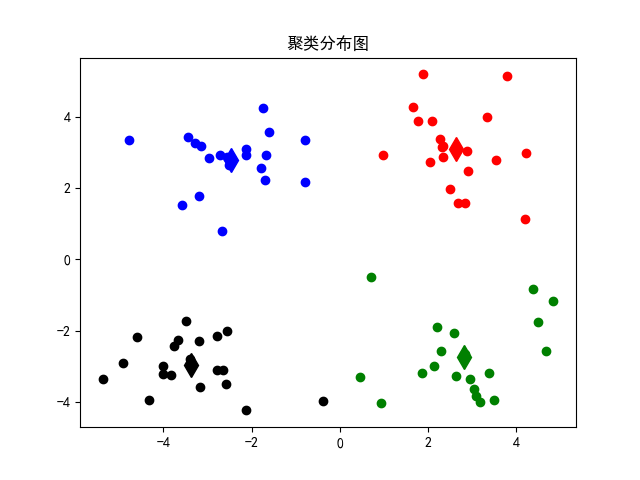k-means测试结果，该算法有效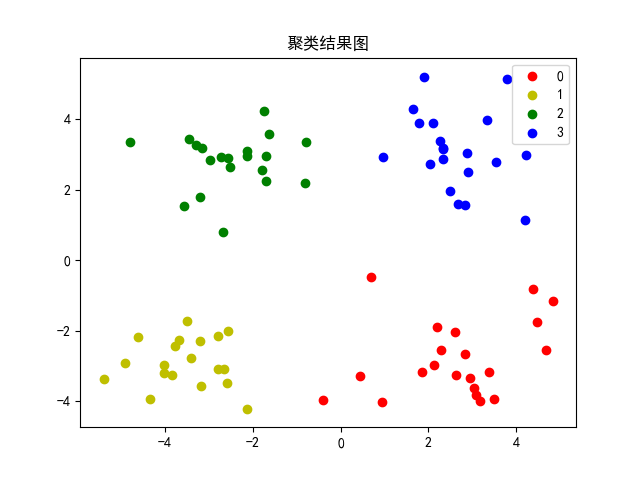AGNES的结果，算法有效
为了比较性能，对大量的点进行实验，表格如下：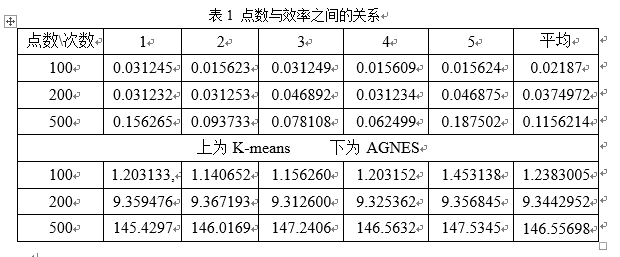具体的算法原理和实验分析见报告内容，最后面提供百度云源码和报告的连接
心得体会
大数据可以说是现在研究十分火热的一个课题，在众多的研究室和科技公司等都是属于一个较为核心的项目。尤其是在当今网络迅速发展的时代，信息就意味着资源，如果能掌握信息就能把握机会。作为大数据分析的关键环节，聚类为分析者提供了效果超凡的数据预处理，使得我们可以发现在数据之下隐藏的逻辑关系与形势。
在众多的聚类算法之中，我们对两个基本的算法K-means和AGNES进行了了解和学习，并实用python语言来实现和对比两者的效果。结果表明，从大数据量的运算来讲，K-means快很多，但AGNES对数据的适用性更高。
在模式识别课程上，学习到了很多有关的人工智能和大数据分析等的知识，可以说获益匪浅。经过动手做实验，更加加深了我对聚类方法的理解，有助于以后更加深入的学习。首先，我要感谢老师耐心细致的授课，对基本概念的讲解十分简明易懂，采用的方式也让人容易理解。其次，感谢我的组员同学们对我实验期间的帮助，共同进步，共同提高。
下载地址：
链接: https://pan.baidu.com/s/1r0MXJSKO5uKq4hWa5IL0Dw 密码: 6sd3


展开全文大数据 python编程 k-means
• K-means聚类算法实验： 通过聚类，了解1999年各个省份的消费水平在国内的情况。 [city.txt 为1999年各个省份的消费水平在国内的情况，数据文件在资源列表下载即可。 #K-means test import numpy as np //①建立...机器学习 pca降维 python
• 对近年来聚类算法的研究现状与新...的聚类算法的聚类情况进行对比分析. 最后通过综合上述两方面信息给出聚类分析的研究热点、难点、不足和有待 解决的一些问题. 上述工作将为聚类分析和数据挖掘等研究提供有益的参考.
• 一、模糊C-means聚类算法 1.简介 模糊c-均值聚类算法 fuzzy c-means algorithm (FCMA)或称（ FCM）。在众多模糊聚类算法中，模糊C-均值（ FCM） 算法应用最广泛且较成功，它通过优化目标函数得到每个样本点对所有...
• 本节对新型密度聚类算法（Clustering by fast search and find of density peaks）的聚类效果及其在相应数据库的性能表现，并与常用的聚类算法K-Means、DBSCAN和谱聚类算法进行了对比分析，所有算法均由...大数据
• 另一方面选择一些典型的聚类算法和一些知名的数据集,主要从正确率和运行效率两个方面进行模拟实验,并分别就同一种聚类算法、不同的数据集以及同一个数据集、不同的聚类算法的聚类情况进行对比分析.最后通过综合上述...
• C均值聚类算法与分级聚类算法的聚类分析 一、实验目的 理解聚类的整体思想，了解聚类的一般方法； 掌握 C-means与分级聚类算法算法思想及原理，并能够熟练运用这些算法进行聚类分析； 能够分析二者的优缺点 二、...python 模式识别
• 层次聚类算法是运行复杂度较高的聚类算法,基于不相似性测度的层次聚类算法不适合稀疏高维数据.结合核函数特点,提出了一种...利用该算法,对稀疏高维数据进行了层次聚类对比,实验结果表明,该算法提高了层次聚类的准确率.
• ## 层次聚类算法

万次阅读 2016-06-05 09:23:29
层次聚类算法是一个应用广泛的算法，小编最近要做对比实验，实现了其中一个版本，为了验证实验效果，结合我国各省会城市之间的距离，对省进行聚类看看效果如何。所有本文从3部分来介绍，首先简介层次聚类算法，然后...
• 聚类算法是我论文实验部分的对比算法，一对比发现，谱聚类真的太强了，聚类效果很好，对不同的数据分布有很强的适应性，速度还快。下文全部参考https://www.cnblogs.com/pinard/p/6221564.html，写得太棒了，自己...
• 利用标准的分类测试集合进行聚类质量的量化评价，选择了k―Means聚类算法、STC（后缀树聚类）算法和基于Ant的聚类算法进行了实验对比实验结果分析表明，STC聚类算法由于在处理文本时充分考虑了文本的短语特性，其...
• 基于群体智能优化算法的图像聚类分析，大多数都采用...在仿真实验中，采用4组数据集对算法进行聚类有效性测试，并将其与4种常用的聚类算法进行对比实验结果表明该算法具有较强的全局搜索能力，稳定性高、聚类效果好。混合编码 量子粒子群
• 为了证明基于混合高斯模型的用电量计量数据聚类算法的集中聚类性能较强，将传统用电量计量数据聚类算法与该算法进行对比实验，实验结果证明该算法的集中聚类性能优于传统用电量计量数据聚类算法，更适用于用电量计量...
• 1 聚类算法总结 常用的聚类算法包括： （1）启发式分割算法：起始确定K个中心点，用距离公式来判断数据点归属，用代价函数（如最小化平方和）评价聚类结果，迭代直至最优，例如：K-Means，K-Medoids。 （2）.
• 实验阶段，采用4组标准数据集对该算法进行了分类实验及有效性测试，并将其与模糊c均值聚类算法及直觉模糊c均值聚类算法的分类效果及运行时间进行对比实验结果充分表明了该算法的有效性及优越性。直觉模糊集 粒子群优化
• ## C均值聚类算法

千次阅读 多人点赞 2019-06-28 14:18:03
聚类算法 1.实验要求： 请使用C均值聚类方法对数据集进行聚类，给每个样本一个类别标签，并画出聚类结果（参考图trainning sample的画法），并与其真实标签（在truelabel.mat中）进行对比，计算聚类的准确率； 3....机器学习
• 文章目录聚类算法1 k-Means算法1.1 基本概念1.2 k-Means算法原理1.3 k-Means算法的可视化演示1.4 实验2 DBSCAN算法2.1 基本概念2.2 DBSCAN算法原理2.3 DBSCAN算法的可视化演示2.4 实验总结 聚类算法 在机器学习中，...机器学习
• 通过在人工数据集上与UCI真实数据集上的实验，将该改进算法与原密度峰值聚类、K-means及DBSCAN算法进行了对比，证明了改进算法能够在密度不均匀数据集上有效完成聚类，能够发现任意形状簇，且在三个聚类性能指标上...
• 论文题目：聚类算法研究 论文来源：聚类算法研究 翻译人：BDML@CQUT实验聚类算法研究 ...另一方面选择一些典型的聚类算法和一些知名的数据集,主要从正确率和运行效率两个方面进行模拟实验,并分别就同一种
• ## 详解DBSCAN聚类算法

千次阅读 2019-04-22 16:21:49
DBSCAN是一种基于密度的聚类算法，它可以在带噪声的数据空间中发现任意形状的类别簇。DBSCAN
• 这节主要讲聚类算法k-means和他的优化算法EM算法机器学习
• 一、数据集描述 二、K-Means算法实现聚类 三、模糊C均值聚类算法实现 四、K-Means算法与模糊C均值聚类算法结果对比 五、优缺点
• 摘要:对近年来聚类算法的研究现状与...的聚类算法的聚类情况进行对比分析.最后通过综合上述两方面信息给出聚类分析的研究热点、难点、不足和有待 解决的一些问题.上述工作将为聚类分析和数据挖掘等研究提供有益的参考.
•Kmeans 机器学习 数据挖掘...